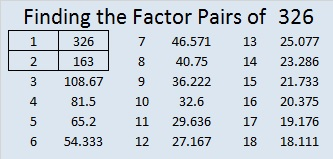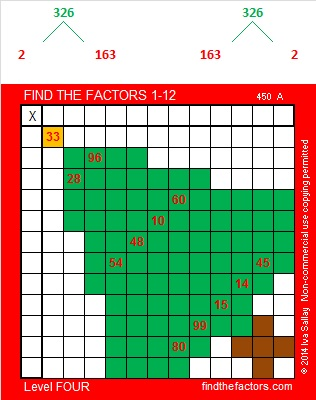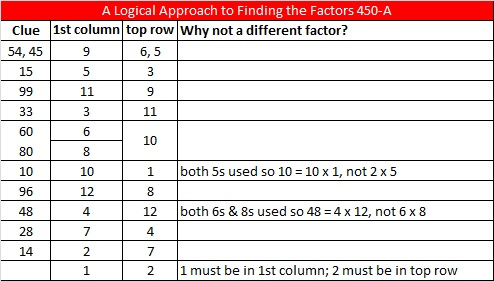# 326 Tiny Christmas Factor Tree

• 326 is a composite number.
• Prime factorization: 326 = 2 x 163
• The exponents in the prime factorization are 1 and 1. Adding one to each and multiplying we get (1 + 1)(1 + 1) = 2 x 2 = 4. Therefore 326 has exactly 4 factors.
• Factors of 326: 1, 2, 163, 326
• Factor pairs: 326 = 1 x 326 or 2 x 163
• 326 has no square factors that allow its square root to be simplified. √326 ≈ 18.055Even though 326 is a three digit number, there is only one way to construct its factor tree, two if you count its mirror image. Either way is illustrated here. Below them is a Christmas factor tree puzzle that is a lot more interesting than the factor tree for 326.Print the puzzles or type the factors on this excel file: 12 Factors 2014-12-15